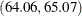### Example 4.9 Computing Confidence Limits for the Mean, Standard Deviation, and Variance

This example illustrates how to compute confidence limits for the mean, standard deviation, and variance of a population. A researcher is studying the heights of a certain population of adult females. She has collected a random sample of heights of 75 females, which are saved in the data set `Heights`:

```data Heights;
label Height = 'Height (in)';
input Height @@;
datalines;
64.1 60.9 64.1 64.7 66.7 65.0 63.7 67.4 64.9 63.7
64.0 67.5 62.8 63.9 65.9 62.3 64.1 60.6 68.6 68.6
63.7 63.0 64.7 68.2 66.7 62.8 64.0 64.1 62.1 62.9
62.7 60.9 61.6 64.6 65.7 66.6 66.7 66.0 68.5 64.4
60.5 63.0 60.0 61.6 64.3 60.2 63.5 64.7 66.0 65.1
63.6 62.0 63.6 65.8 66.0 65.4 63.5 66.3 66.2 67.5
65.8 63.1 65.8 64.4 64.0 64.9 65.7 61.0 64.1 65.5
68.6 66.6 65.7 65.1 70.0
;
```

The following statements produce confidence limits for the mean, standard deviation, and variance of the population of heights:

```title 'Analysis of Female Heights';
ods select BasicIntervals;
proc univariate data=Heights cibasic;
var Height;
run;
```

The CIBASIC option requests confidence limits for the mean, standard deviation, and variance. For example, Output 4.9.1 shows that the 95% confidence interval for the population mean is. The ODS SELECT statement restricts the output to the BasicIntervals table; see the section ODS Table Names.

The confidence limits in Output 4.9.1 assume that the heights are normally distributed, so you should check this assumption before using these confidence limits. See the section Shapiro-Wilk Statistic for information about the Shapiro-Wilk test for normality in PROC UNIVARIATE. See Example 4.19 for an example that uses the test for normality.

Output 4.9.1: Default 95% Confidence Limits

 Analysis of Female Heights

The UNIVARIATE Procedure
Variable: Height (Height (in))

Basic Confidence Limits Assuming Normality
Parameter Estimate 95% Confidence Limits
Mean 64.56667 64.06302 65.07031
Std Deviation 2.18900 1.88608 2.60874
Variance 4.79171 3.55731 6.80552

By default, the confidence limits produced by the CIBASIC option produce 95% confidence intervals. You can request different level confidence limits by using the ALPHA= option in parentheses after the CIBASIC option. The following statements produce 90% confidence limits:

```title 'Analysis of Female Heights';
ods select BasicIntervals;
proc univariate data=Heights cibasic(alpha=.1);
var Height;
run;
```

The 90% confidence limits are displayed in Output 4.9.2.

Output 4.9.2: 90% Confidence Limits

 Analysis of Female Heights

The UNIVARIATE Procedure
Variable: Height (Height (in))

Basic Confidence Limits Assuming Normality
Parameter Estimate 90% Confidence Limits
Mean 64.56667 64.14564 64.98770
Std Deviation 2.18900 1.93114 2.53474
Variance 4.79171 3.72929 6.42492

For the formulas used to compute these limits, see the section Confidence Limits for Parameters of the Normal Distribution.

A sample program for this example, uniex07.sas, is available in the SAS Sample Library for Base SAS software.